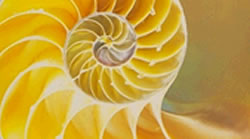You may also likeFixing It

A and B are two fixed points on a circle and RS is a variable diamater. What is the locus of the intersection P of AR and BS?OK! Now Prove It

Make a conjecture about the sum of the squares of the odd positive integers. Can you prove it?Summats Clear

Find the sum, f(n), of the first n terms of the sequence: 0, 1, 1, 2, 2, 3, 3........p, p, p +1, p + 1,..... Prove that f(a + b) - f(a - b) = ab.

Fibonacci Factors

Age 16 to 18Challenge LevelIn the Fibonacci sequence each term is the sum of the two terms before it:

1, 1, 2, 3, 5, 8, 13, 21, 34, 55, 89, 144...

Where do the even numbers come in the sequence?

Is there a pattern? Why?

Which Fibonacci numbers are divisible by 3?

Prove general results for the occurrence of even Fibonacci numbers in the sequence and for the occurrence of multiples of 3.